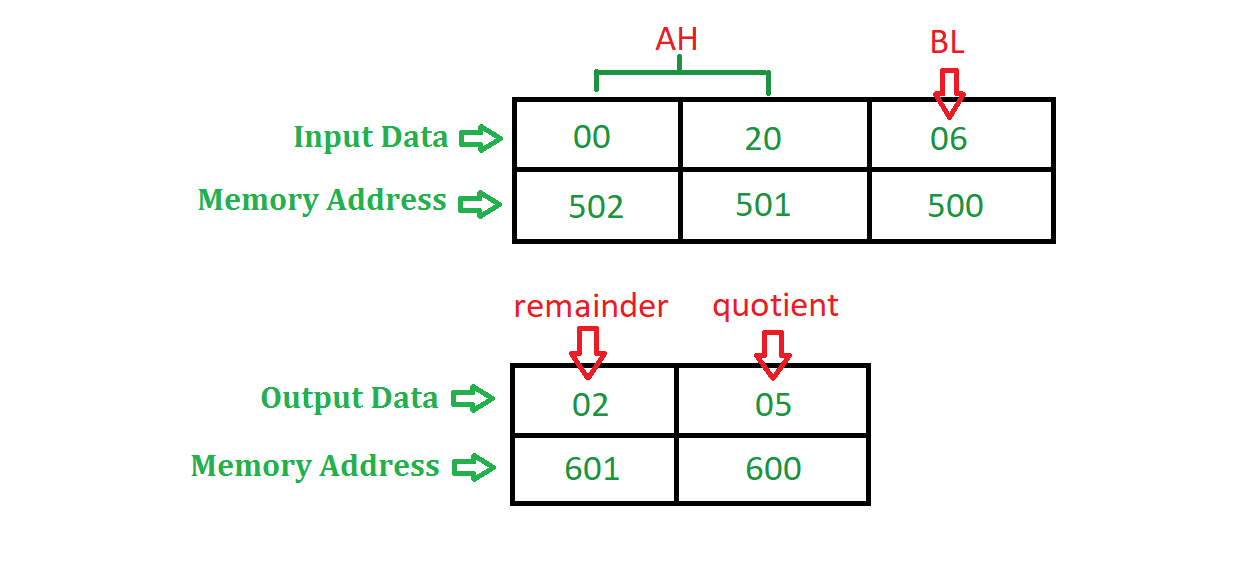# 8086 program to divide a 16 bit number by an 8 bit number

Problem – Write an assembly language program in 8086 microprocessor to divide a 16 bit number by an 8 bit number.

Example –Algorithm –

1. Assign value 500 in SI and 600 in DI
2. Move the contents of [SI] in BL and increment SI by 1
3. Move the contents of [SI] and [SI + 1] in AX
4. Use DIV instruction to divide AX by BL
5. Move the contents of AX in [DI].
6. Halt the program.

Assumption – Initial value of each segment register is 00000.

Calculation of physical memory address –
Memory Address = Segment Register * 10(H) + offset,
where Segment Register and Offset is decided on the basis of following table.

OPERATIONS SEGMENT REGISTER OFFSET
Instruction fetching Code Segment Instruction Pointer
Data operation Data Segment Base Register [BX], Displacement [DISP]
Stack operation Stack Segment Stack Pointer (SP), Base Pointer (BP)
String as a source Data Segment Source Indexed (SI)
String as a destination Extra Segment Destination Indexed (DI)

Program –

0400 MOV SI, 500 SI <- 500
0403 MOV DI, 600 DI <- 600
0406 MOV BL, [SI] BL <- [SI]
0408 INC SI SI <- SI + 1
0409 MOV AX, [SI] AX <- [SI]
040B DIV BL AX <- AX / BL
040D MOV [DI], AX [DI] <- AX
040F HLT End of program

Explanation – Registers used AX, BL, SI, DI

1. MOV SI, 500 assigns 500 to SI
2. MOV DI, 600 assigns 600 to DI
3. MOV BL, [SI] moves the content of [SI] to BL register i.e. value of divisor will be stored in BL
4. INC SI increment the content of SI by 1
5. MOV AX, [SI] moves the content of [SI] and [SI + 1] to AX register i.e. value of dividend will be stored in AX
6. DIV BL divide the content of AX by BL, after execution of this instruction the quotient get stored in AL and remainder in AH
7. MOV [DI], AX moves the content of AX to [DI]
8. HLT stops executing the program and halts any further execution

Don’t stop now and take your learning to the next level. Learn all the important concepts of Data Structures and Algorithms with the help of the most trusted course: DSA Self Paced. Become industry ready at a student-friendly price.

My Personal Notes arrow_drop_upCheck out this Author's contributed articles.

If you like GeeksforGeeks and would like to contribute, you can also write an article using contribute.geeksforgeeks.org or mail your article to contribute@geeksforgeeks.org. See your article appearing on the GeeksforGeeks main page and help other Geeks.

Please Improve this article if you find anything incorrect by clicking on the "Improve Article" button below.